Get Started »

# Group Theory

SymmetricGroup, AlternatingGroup, DihedralGroup and many other named groups are built-in symbols.

Get a list of group elements:

 In:=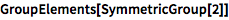⨯ `GroupElements[SymmetricGroup]`
 Out=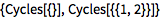Determine the generators of a group:

 In:=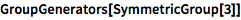⨯ `GroupGenerators[SymmetricGroup]`
 Out=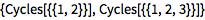Create a permutation group from two generators:

 In:=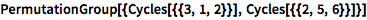⨯ `PermutationGroup[{Cycles[{{3, 1, 2}}], Cycles[{{2, 5, 6}}]}]`

Compute its order:

 In:=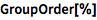⨯ `GroupOrder[%]`
 Out=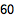Show the multiplication table for a group:

 In:=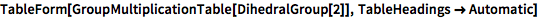⨯ ```TableForm[GroupMultiplicationTable[DihedralGroup], TableHeadings -> Automatic]```
 Out=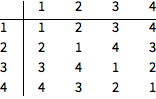Visualize it with a Cayley graph:

 In:=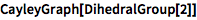⨯ `CayleyGraph[DihedralGroup]`
 Out=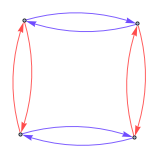QUICK REFERENCE: Group Theory `»`